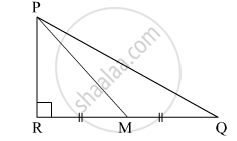Share

# In the Given Figure, M is the Midpoint of Qr. ∠Prq = 90°. Prove That, Pq2 = 4pm2 – 3pr2 - Geometry

ConceptPythagoras Theorem

#### Question

In the given figure, M is the midpoint of QR. ∠PRQ = 90°. Prove that, PQ= 4PM– 3PR2#### Solution

According to Pythagoras theorem,

In ∆PRM

${PR}^2 + {RM}^2 = {PM}^2$
$\Rightarrow {RM}^2 = {PM}^2 - {PR}^2 . . . \left( 1 \right)$

In ∆PRQ

${PR}^2 + {RQ}^2 = {PQ}^2$
$\Rightarrow {PQ}^2 = {PR}^2 + \left( RM + MQ \right)^2$
$\Rightarrow {PQ}^2 = {PR}^2 + \left( RM + RM \right)^2$
$\Rightarrow {PQ}^2 = {PR}^2 + \left( 2RM \right)^2$
$\Rightarrow {PQ}^2 = {PR}^2 + 4 {RM}^2$
$\Rightarrow {PQ}^2 = {PR}^2 + 4\left( {PM}^2 - {PR}^2 \right) \left( \text{from} \left( 1 \right) \right)$
$\Rightarrow {PQ}^2 = {PR}^2 + 4 {PM}^2 - 4 {PR}^2$
$\Rightarrow {PQ}^2 = 4 {PM}^2 - 3 {PR}^2$

Hence, PQ= 4PM– 3PR2.

Is there an error in this question or solution?

#### Video TutorialsVIEW ALL 

Solution In the Given Figure, M is the Midpoint of Qr. ∠Prq = 90°. Prove That, Pq2 = 4pm2 – 3pr2 Concept: Pythagoras Theorem.
S